## Thursday, 7 August 2014

### chapter 7- Summary of Probability Concepts

Let us summarize what concepts we've encountered up to now in one form or the other. Note: this summary is exceptionally important. Make sure that you have a good idea about what all’s involved.
(i) Outcome
The result of a random experiment . For example, in the tossing of a coin, obtaining “Heads” is a possible outcome.
(ii) Equally likely
Two outcomes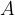$A$ and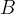$B$ of an experiment can be said to be equally likely when there is no evident reason to favor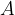$A$ over$B$ or viceversa. To make the idea more concrete, you can say that as you repeat the experiment an indefinitely large number of times, the relative occurrence of$A$ and$B$ will be equal. For example, if you toss a fair coin an indefinitely large number of times, the relative occurrence of both Heads and Tails will be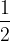$\dfrac{1}{2}$. Similarly, if a die is rolled an indefinitely large number of times, each of the six faces will have a relative occurrence of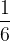$\dfrac{1}{6}$.
(iii) Event
An event is a set of outcomes. Thus, an event can be viewed as a subset of the universe of all outcomes of the experiment, which is termed as the sample space of the experiment.
For example, in drawing a card from a well-shuffled deck of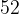$52$ cards at random, the sample space is of size$52$. The event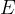$E$ defined as$E$ : The card drawn is red is a set of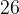$26$ outcomes.
The event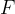$F$ defined as$F$ : The card drawn is a king
is a set of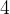$4$ outcomes.
The event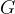$G$ defined as$G$ : The card drawn is the Ace of spades
is a set of only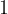$1$ outcome, and is thus an elementary event, whereas$E$ and$F$are compound events.
(iv) Probability
If all outcomes in an experiment are equally likely (like in tossing a fair coin, rolling a fair die, drawing a card at random from a well-shuffled deck), then the probability of occurrence of an event$E$ is simply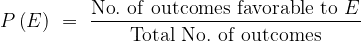$P\left( E \right)\,\, = \,\,\dfrac{{{\rm{No}}{\rm{.}}\,\,{\rm{of}}\,\,{\rm{outcomes}}\,\,{\rm{favorable}}\,\,{\rm{to}}\,\,E}}{{{\rm{Total}}\,\,{\rm{No}}{\rm{.}}\,\,{\rm{of}}\,\,{\rm{outcomes}}}}$
Note that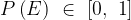$P\left( E \right)\,\, \in \,\,\left[ {0,\,\,1} \right]$
(Note also that probability defined this way is not applicable to situations like weather prediction, which use advanced probabilistic models).
(v) Events as sets
Since events are sets of outcomes, set operations can be defined for events. Let$E$ and$F$ be two events associated with a random experiment. In general, we have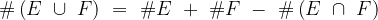$\# \left( {E\,\, \cup \,\,F} \right)\,\, = \,\,\# E\,\, + \,\,\# F\,\,-\,\,\# \left( {E\,\, \cap \,\,F} \right)$
In case of mutually exclusive events, this reduces to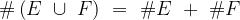$\# \left( {E\,\, \cup \,\,F} \right)\,\, = \,\,\# E\,\, + \,\,\# F\,$
(vi) Conditional Probability
If$E$ and$F$ are two events, then the probability of$E$ occurring given that$F$ has already occurred is termed the conditional probability of$E$ given$F$, and is written as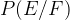$P(E/F)$. We have,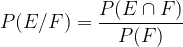$P(E/F) = \dfrac{{P(E \cap F)}}{{P(F)}}$
(vii) Independent events
If$E$ and$F$ are independent events, then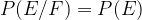$P(E/F) = P(E)$ and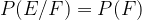$P(E/F) = P(F)$ which implies that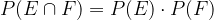$P(E \cap F) = P(E) \cdot P(F)$
This basically means that the probability of$E$ occurring is not affected by the occurrence or non-occurrence of$F$, and vice-versa.
(viii) Difference between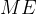$ME$ and independent events
If$E$ and$F$ are two non-impossible events, then
 if$E$ and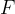$F$ are independent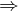$\Rightarrow$ they are not$ME$ if$E$ and$F$ are$ME$$\Rightarrow$ they are not independent